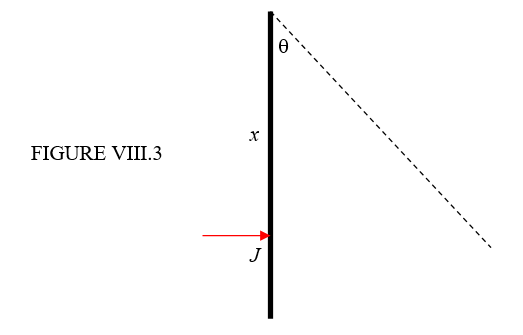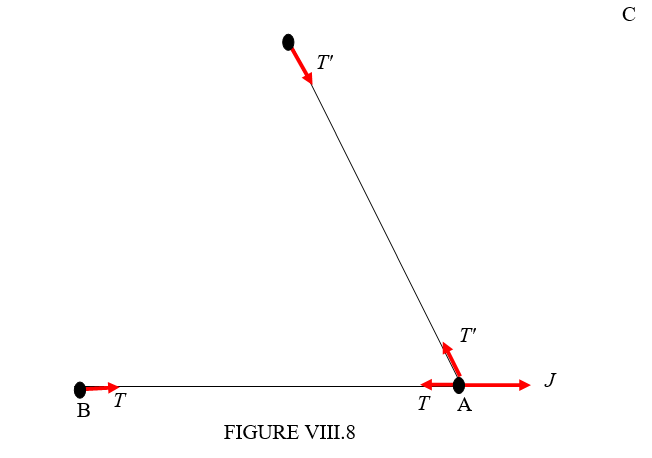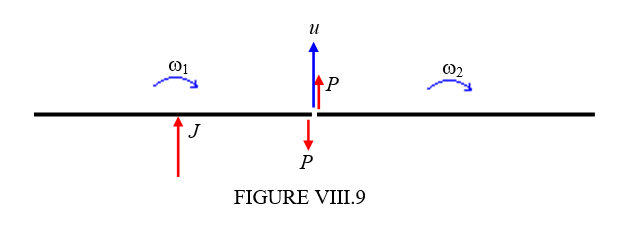$$\require{cancel}$$

# 8.2: Problem

•• Contributed by Jeremy Tatum
• Emeritus Professor (Physics & Astronomy) at University of Victoria

In this section I offer a set of miscellaneous problems. In a typical problem it is assumed that an impulsive force or torque acts for only a very short time. By "a very short" time, I mean that the time during which the force or torque acts is very small or is negligible compared with other times that might be involved in the problem. For example, if a golf club hits a ball, the club is in contact with the ball for a time that is negligible compared with the time in which the ball is in the air. Or if a pendulum is subjected to an impulsive torque, the time during which the impulsive torque is applied is negligible compared with the period of the pendulum.

In many problems, you will be told that a body is subjected to an impulse $$J$$. The easiest way to interpret this is to say that the linear momentum of the body suddenly changes by $$J$$. Or you may be told that the body is subjected to an impulsive torque $$K$$. The easiest way to interpret this is to say that the angular momentum of the body suddenly changes by $$K$$.

In some of the problems, for example the first one, the body concerned is freely hinged about a fixed point; that is, it can freely rotate about that point.

Before giving the first problem, here is a little story. One of the most inspiring lectures I remember going to was one given by a science educator. She complained that a professor, instead of inspiring his students with a love and appreciation of the great and profound ideas of science and civilization, "infantalized" the class with a tiresome insistence that the class use blue pencils for velocity vectors, green for acceleration, and red for forces. I recognized immediately that this was a great way of imparting to students an appreciation of the profound ideas of physics, and I insisted on it with my own students ever since. In some of the following drawings I have used this colour convention, though I don't know whether your computer will reproduce the colours that I have used. In any case, I strongly recommend that you use the colour convention so deprecated by the educator if you want to understand the great ideas of civilization, such as the ideas of impulsive forces.

Exercise $$\PageIndex{1}$$In Figure VIII.2, a body is free to rotate about a fixed axis O. The centre of mass of the body is at C. The distance OC is h. The body is struck with a force of impulse $$J$$ at A, such that OA = $$x$$. The mass of the body is $$m$$. Its rotational inertia about C is $$mk^{2}$$, and its rotational inertia about O is $$m(k^{2}+h^{2})$$.

As a result of the blow, the body will rotate with angular speed w and the centre of mass will move forward with linear speed hw. One of the questions in this problem is to calculate $$\omega$$

The body will also push with an impulsive force against the axis at O. It is not immediately obvious whether the body will push upwards against the axis in the same direction as $$J$$, or whether the left hand end of the body will swing downwards and the body will push downwards on the axis. You will probably agree that if A is very close to O, the body will push upwards on the axis, but if A is near the right hand end, the body will push downward on the axis. In the Figure, I am assuming that the body pushes upwards on the axis; the axis therefore pushes downwards on the body, with a force of impulse $$P$$, and what the Figure shows is the two impulsive forces that act on the body. The second question to be asked in this problem is to find $$P$$ in terms of $$J$$ and $$x$$.

If we are right in our intuitive feeling that $$P$$ acts upwards or downwards according to the position of A - i.e. on where the body is struck - there is presumably some position of A such that the reactive impulse of the axis on the body is zero. Indeed there is, and the position of A that gives rise to zero reactive impulse at A is called the centre of percussion, and a third question in this problem is to find the position of the centre of percussion. Where on the bat should you hit the baseball if you want zero impulsive reaction on your wrists? Where should you position a doorstop so as to result in zero reaction on the door hinges? Never let it be said that theoretical physics does not have important practical applications. The very positioning of a door-stop depends on a thorough understanding of the principles of classical mechanics.

The net upward impulse is $$J - P$$, and this results in a change in linear momentum $$mh\omega$$:

$J - P = mh\omega \tag{8.2.1}\label{eq:8.2.1}$

The impulsive torque about O is $$Jx$$, and this results in a change in angular momentum $$I\omega$$; that is to say $$m(k^{2}+h^{2})\omega$$:

$Jx=m(k^{2}+h^{2})\omega. \tag{8.2.2}\label{eq:8.2.2}$

These two equations enable us to solve for the two unknowns $$\omega$$ and $$P$$. Indeed, Equation $$\ref{eq:8.2.2}$$ gives us $$\omega$$ immediately, and elimination of $$\omega$$ between the two equations gives us $$P$$:

$P = J\left(1-\frac{xh}{k^{2}+h^{2}}\right). \tag{8.2.3}\label{eq:8.2.3}$

If the right hand side of Equation $$\ref{eq:8.2.3}$$ is positive, then Figure VIII.2 is correct: the axis pushes down on the body, and the body pushes upwards on the axis. That is, $$P$$ acts downwards if $$x<\frac{k^{2}+h^{2}}{h}$$, and upwards if $$x>\frac{k^{2}+h^{2}}{h}$$. The position of the centre of percussion is $$x=\frac{k^{2}+h^{2}}{h}$$.

If the body is a uniform rod of length $$l$$, O is at one end of the rod, then $$k^{2}=\frac{1}{12}l^{2}$$ so that, in this case, $$x=\frac{2}{3}l$$. This is where you should position a door-stop. It is also where you should hit a baseball with the bat - if the bat is a uniform rod. However, I admit to not knowing a great deal about baseball bats, and if such a bat is not a uniform rod, but is, for example, thicker and heavier at the distal than the proximal end, the centre of percussion will be further towards the far end.

Exercise $$\PageIndex{2}$$A heavy rod, of mass $$m$$ and length $$2l$$, hangs freely from one end. It is given an impulse $$J$$ as shown at a point at a distance $$x$$ from the upper end. Calculate the maximum angular height through which the rod rises.

We can use Equation $$\ref{eq:8.2.2}$$ to find the angular speed $$\omega$$ immediately after impact. In this equation, $$m(k^{2}+h^{2})$$ is the rotational inertia of the rod about its end, which is $$\frac{4ml^{2}}{3}$$, so that

$$\omega = \frac{3Jx}{4ml^{2}}$$.

The kinetic energy immediately after impact is $$\frac{1}{2}\cdot\frac{4}{3}ml^{2}\cdot\omega^{2}$$ and we have to equate this to the subsequent gain in potential energy $$mgl(1-\cos\theta)$$.

Thus

$$\cos\theta=1-\frac{2l\omega^{2}}{3g}=1-\frac{2J^{2}x^{2}}{8gm^{2}l^{3}}$$.

To get the rod to swing through 180o, the angular impulse applied must be

$$Jx=4ml\sqrt{\frac{gl}{3}}$$.

Exercise $$\PageIndex{3}$$A uniform rod of mass $$m$$ and length $$2l$$ is freely hinged at one end O. A mass $$cm$$ (where $$c$$ is a constant) is attached to the rod at a distance $$x$$ from O. An impulse $$J$$ is applied to the other end of the rod from O. Where should the mass $$cm$$ be positioned if the linear speed of the mass $$cm$$ immediately after the application of the impulse is to be greatest?

The angular impulse about O is $$2lJ$$. The rotational inertia about O is $$\frac{4}{3}ml^{2}+cmx^{2}$$. If w is the angular speed immediately after the blow, the angular momentum is $$(\frac{4}{3}ml^{2}+cmx^{2})\omega$$. If we equate this to the impulse, we find

$$\omega=\frac{6lJ}{m(4l^{2}+3cx^{2})}$$.

The linear speed of the mass $$cm$$ is $$x$$ times this, or $$\frac{6lJ}{m(4l^{2}+3cx^{2})}$$. By calculus, this is greatest when $$x=\frac{2l}{\sqrt{3c}}$$.

Exercise $$\PageIndex{4}$$A uniform rod is of mass $$m$$ and length $$2l$$. An impulse $$J$$ is applied as shown at a distance $$x$$ from the mid-point of the rod. P is a point at a distance y from the mid-point of the rod. Does P move forward or backward? Which way does A move?

The first thing we can do is to find the linear speed $$u$$ of the centre of mass of the rod and the angular speed $$\omega$$ of the rod. We do this by equating the impulse to the increase in linear momentum and the moment of the impulse (i.e. the angular impulse) to the increase in angular momentum:

$$J = mu$$

and

$$Jx=\frac{1}{3}ml^{2}\omega$$.

Thus we know both $$u$$ and $$\omega$$.

The forward velocity of P is $$u +y\omega$$. That is to say $$\frac{J}{m}+\frac{3Jxy}{ml^{2}}$$. This is positive if $$y>-\frac{l^{2}}{3x}$$ but negative otherwise. For the point A, $$y=-l$$, so that A will move forward if $$x<\frac{l}{3}$$, and it will move backwards otherwise.

Exercise $$\PageIndex{5}$$A spherical planet, mass $$m$$, radius $$a$$, is struck by an asteroid with an impulse $$J$$ as shown, the impact parameter being $$x$$. P is a point on the diameter, a distance $$y$$ from the centre of the planet. Does P move forward or backward? Which way does A move?

As in the previous problem, we can easily find $$u$$ and $$\omega$$:

$$J = mu$$

and

$$Jx = \frac{2}{5}ma^{2}\omega$$.

The forward velocity of P is $$u+y\omega$$. That is to say $$\frac{J}{m}(1+\frac{5xy}{2a^{2}})$$. This is positive if $$y>-\frac{2a^{2}}{5x}$$ but negative otherwise. For the point A, $$y=-a$$, so that A will move forward if $$x<\frac{2a}{5}$$, and it will move backward otherwise. That is to say A will move backwards if the blow is more that 70% of the way from A to B.

Exercise $$\PageIndex{6}$$

A hoop, radius $$a$$, mass $$m$$, moving at speed $$v$$, hits a kerb of height $$h$$ as shown. Will it mount the kerb, or will it fall back?

The only impulse acts at the point A. The moment of the impulse about A is therefore zero, and therefore there is no change in angular momentum with respect to the point A.

Before impact, the angular momentum with respect to the point A is

$$mva+mv(a-h)=mv(2a-h)$$.

Let $$\omega$$ be the angular speed about A after impact. The angular momentum about A is then $$2ma^{2}\omega$$. These are equal, so that

$$\omega=\frac{(2a-h)v}{2a^{2}}$$

If it is to mount the kerb, the new kinetic energy $$\frac{1}{2}(2ma^{2})\omega^{2}$$ must be greater than the potential energy that is to be overcome, $$mgh$$.

Therefore

$$v>\frac{2a\sqrt{gh}}{2a-h}$$.

Exercise $$\PageIndex{7}$$Three equal particles, A, B and C, each of mass m, are joined by light inextensible strings as shown, the angle BAC being 60o. A is given a sharp tug of impulse $$J$$ as shown. Find the initial velocities of the particles and the initial tensions in the strings.

Let the initial tensions in BA and AC be $$T$$ and $$T'$$ respectively.

Let the initial velocity of A be $$u\bf{i}+v\bf{j}$$.

Then the initial velocity of B is $$u\bf{i}$$

and the initial velocity of C is $$\frac{1}{2}(u-\sqrt{3v})$$ towards A.

The equations of impulsive motion are:

For B:

$$mu=T$$

For C:

$$\frac{1}{2}(u-\sqrt{3v})=T'$$

For A:

$$mu=J-T-\frac{1}{2}T'$$

$$mv=\frac{1}{2}\sqrt{3}T'$$

The solutions of these equations are:

$$u=\frac{7J}{15m}$$, $$v=\frac{\sqrt{3}J}{15m}$$,

$$T=\frac{7J}{15}$$, $$T'=\frac{2J}{15}$$.

Exercise $$\PageIndex{8}$$Two rods, each of mass $$m$$ and length $$2l$$, are freely jointed as shown. One of them is given an impulse $$J$$ as shown. What happens then is that the end of one rod gives the end of the other an impulsive kick $$P$$, and the other gives the one an equal kick in the opposite direction. The centre of mass of the system moves forward with speed $$u$$ and the two rods rotate with angular speeds $$\omega_{1}$$ and $$\omega_{2}$$. The problem is to determine $$P$$, $$u$$, $$\omega_{1}$$ and $$\omega_{2}$$.

The equations of impulsive motion are:

LH rod, translation:

$$J-P=m(u+l\omega_{1})$$,

RH rod, translation:

$$P=m(u+l\omega_{2})$$

LH rod, rotation:

$$Pl=\frac{1}{3}ml^{2}\omega_{1}$$

RH rod, rotation:

$$Pl=\frac{1}{3}ml^{2}\omega_{1}$$

The solutions to these equations are:

$$u=\frac{J}{2m}$$,

$$\omega_{1}=\omega_{2}=\frac{3J}{8ml}$$,

$$P=\frac{J}{8}$$.

Exercise $$\PageIndex{9}$$Two rods, each of mass $$m$$ and length $$2l$$, are freely jointed initially at right angles. They are dropped on to a smooth horizontal table and strike it with speed $$V$$. Find the rate $$\dot{\theta}$$ at which the rods splay apart immediately after impact.

We consider the dynamics of the right hand rod. On impact, it experiences a vertical impulse $$J$$ at its lower end, and it experiences a horizontal impulse $$P$$ (from the other rod) at its upper end. Immediately after impact, let the components of the velocity of the centre of mass of the right hand rod be $$u$$ and $$v$$, and the angular speed of the rod is the required quantity $$\dot{\theta}$$.

From geometry, we have

$$x=l\sin\theta$$ and $$y=l\cos\theta$$

and hence

$$u=\dot{x}=l\cos\theta\dot{\theta}=\frac{l\dot{\theta}}{\sqrt{2}}$$ and $$v=-\dot{y}=l\sin\theta\dot{\theta}=\frac{l\theta}{\sqrt{2}}$$.

The impulsive equations of motion are

$$P=mu$$

and

$$J=m(V-v)$$.

After that, some algebra results in

$$\dot{\theta}=\frac{\sqrt{18}V}{8l}$$.

Exercise $$\PageIndex{10}$$A square plate is spinning about a vertical diameter at angular speed $$\omega_{1}$$. It strikes a fixed obstacle at the corner A, so that it subsequently spins about a vertical axis through A at angular speed $$\omega_{2}$$. Find $$\omega_{2}$$.

Since the impulse is at A, the moment of the impulse about A is zero, so that angular momentum about A is conserved. The relevant moments of inertia can be calculated from the information in Chapter 2, and hence we obtain

$$\frac{1}{3}ma^{2}\omega_{1}=\frac{7}{3}ma^{2}\omega_{2}$$.
$$\omega_{2}=\frac{\omega_{1}}{7}$$.